# DMelt:AI/1 Backpropagation Neural Net

Limitted access. First login to DataMelt if you are a full DataMelt member. Then login to HandWiki as a user.

## Backpropagation

This section devoted to one of the most popular neural net: backpropagation. Read Backpropagation article.

## Neural Network using Python

The best way to get started with Neural Networks is to a look at a simply Python code which implements a back propagation neural net. In your DataMelt installation, look at the Python file:

Go to "Tools" and then "Online examples": Artificial Intelligence/neural net/neural_net_bpnn.py

Open it with DataMelt IDE and study it - it is simple and well documented. It is self-contained and does not require Java libraries (so you can modify and rerun it at your convenience). This code creates a neural network with two input, two hidden, and one output nodes. The training sample is given by the matrix, where the first 2 columns are input, and the last column is output.

[[0,0], 0]],
[[0,1], ],
[[1,0], ],
[[1,1], ]

Open it with DataMelt IDE and run it (press [F8]). You will see the output like this:

error 0.849818
error 0.057854
error 0.003672
error 0.001686
error 0.001081
error 0.000792
error 0.000630
error 0.000527
error 0.000445
error 0.000384
[0, 0] -> [-0.021372111181607808]
[0, 1] -> [0.9824168796884087]
[1, 0] -> [0.9822863753023391]
[1, 1] -> [0.022461131355512465]

The output of this scripts gives the training error, and the last lines are the predictions after the training.

## Neural Network using Java

DataMelt contains several powerful Java libraries for unsupervised learning procedures. Unlike Python, libraries implemented in Java is by a factor 10-20 faster. Let us show how to use Jython (or any Java scripting language) to create and run a backpropogation network. This library uses the Encog engine from Heaton Research.

Below we show a simple neural net which reads CSV file, train network using 4-hiden layers and validate it. The input file looks like this:

 0,0,0
1,0,1
0,1,1
1,1,0


Here is a simple 5-line code which performs neural net training.

This example uses several important Java classes:

Now we will extend this example by putting a few more lines:

1. Show the network structure in a separate window
2. Build network analyser to extract weights, data etc.
3. Save the network in a separate file and restore it

The script which does all of this is shown below:

This script generates a pop-up JFrame with the network structure: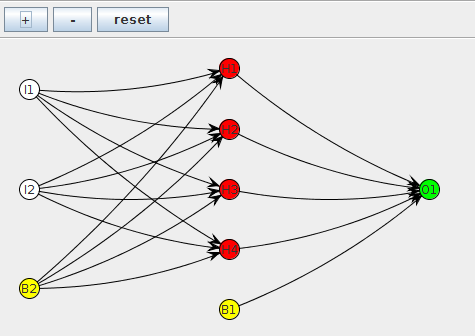## Neural Network scripting

This time we will customize the network. Instead of using predefined factories, we build actual objects and redefine the activation functions. We also use a script to input data.

As before, we will use a simple input with 2 inputs, which will look like this:

XOR_INPUT =  [ [ 0.0, 0.0 ], [1.0, 0.0], [0.0, 1.0], [1.0, 1.0]] # 2 inputs
XOR_IDEAL =  [ [ 0.0 ], [1.0], [1.0], [0.0]]                     # output

Here is Java code which performs backpropogation network analysis:

The same script will print the result of training:

Epoch # 10  Error: 0.238874112686
Epoch # 20  Error: 0.195396962817
Epoch # 30  Error: 0.126547597192
Epoch # 40  Error: 0.0592342636102
Epoch # 50  Error: 0.0286886069541
Epoch # 60  Error: 0.0100759401001

-> Test Trained Neural Network
0.0 , 0.0 , actual= 0.112184658782 ,ideal= 0.0
1.0 , 0.0 , actual= 0.958848378144 ,ideal= 1.0
0.0 , 1.0 , actual= 0.902418114956 ,ideal= 1.0
1.0 , 1.0 , actual= 0.0811959953326 ,ideal= 0.0

As you can see, the predicted values are rather close to the expected.

## Neural Networks using the neuroph packag

You can build and train neural nets using the neuroph package.org/neuroph/nnet/MultiLayerPerceptron. This is Java implementation and should be rather fast. Look at a simple example for multi-layer perceptron for the XOR problem:

and run it inside the DataMelt. It trains and then check th result of the run. DataMelt also includes a similar example but written in Java.

## Backpropogation based on HNeuralNet

Below we will show a simple example how to use neural networks using the classHNeuralNet which is designed for backpropogation neural networks. This library uses the Encog engine from Heaton Research, but restructured for simple scripting using Jython, BeanShell or Groovy or JRuby.

First, let us create a file with our input data which will be analyzed later using a neural network.

Our data structure is represented in the form of dictionary, with two string keys: 'input' (represented by PND array) and 'output' (also represented by a PND array), but consists only one number. Our output is a function of 4 random inputs. The goal of our neural net is to rediscover the relation between 5 inputs and the output, pretending that we know nothing about the such dependence.

Below we analyze this data in the file data.ser using a back propogation artificial neural network. The goal is make predictions on the output value knowing the input variables.

The principle of back propagation is actually quite easy to understand. The basic steps are:

1. Initialize the network with small random weights
2. Present an input pattern to the input layer of the network
3. Feed the input pattern forward through the network to calculate its activation value
4. Take the difference between desired output and the activation value to calculate the network’s activation error
5. Adjust the weights feeding the output neuron to reduce its activation error for this input pattern
6. Propagate an error value back to each hidden neuron that is proportional to their contribution of the network’s activation error.
7. Adjust the weights feeding each hidden neuron to reduce their contribution of error for this input pattern.
8. Repeat steps 2 to 7 for each input pattern in the input collection.
9. Repeat step 8 until the network is suitably trained.

For such iterations, each pattern is presented in turn, and the network adjusted slightly, before moving on to the next pattern. The magic really happens in step 6, which determines how much error to feed back to each hidden neuron. Once the error value has been established, training can continue.

A next step is to build a backpropogation artificial neural network with 5 inputs, 3 hidden layers and one output.

This script builds this topology for neural net: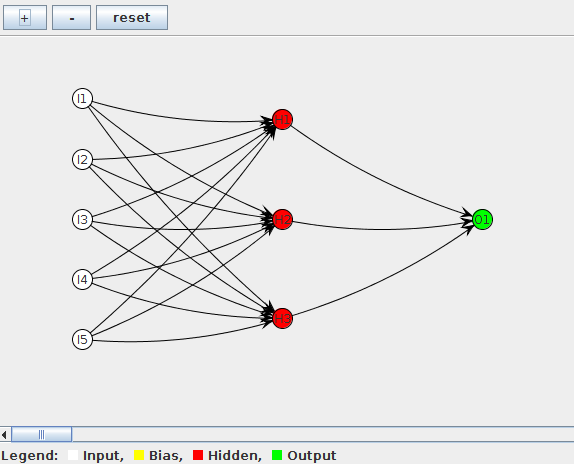For convenience, we randomize inputs and save it into a file "test.eg". The output of this script is shown here: The output file "test.eg" defines neural net; just open this file in any editor and you will find a simple XML definitions of our neural net.

You can find details of the classHNeuralNet here. Once the neural net is saved, one can restore it an any time:

from jhplot import *
net = HNeuralNet()
net.read("test.eg","myNN")  # the name myNN should be the same as in the example above
net.showNetwork()

Now we have prepared data and NN. Next step is to train the NN using the input data. It should be noted that it is desirable to rescale the input values so they will be between [-1,1]. This is explained in more details in the book. Here we will just use the original data for simplicity.

## Neural Net with input CSV data

In this example we build a neural net using CSV input data as shown below. First 2 columns are input, while the last column is output.

0.10, 0.03, 0
0.11, 0.11, 0
0.11, 0.82, 0
0.13, 0.17, 0
0.20, 0.81, 0
0.21, 0.57, 1
0.25, 0.52, 1
0.26, 0.48, 1
0.28, 0.17, 1
0.28, 0.45, 1
0.37, 0.28, 1
0.41, 0.92, 0
0.43, 0.04, 1
0.44, 0.55, 1
0.47, 0.84, 0
0.50, 0.36, 1
0.51, 0.96, 0
0.56, 0.62, 1
0.65, 0.01, 1
0.67, 0.50, 1
0.73, 0.05, 1
0.73, 0.90, 0
0.73, 0.99, 0
0.78, 0.01, 1
0.83, 0.62, 0
0.86, 0.42, 1
0.86, 0.91, 0
0.89, 0.12, 1
0.95, 0.15, 1
0.98, 0.73, 0


In the code below we read this input file and perform backpropogation. The the script shows the structure of neural net, errors as a function of the epoch and the predicted values.

The code generates a neural net as shown here:

## Neural Network for predictions

In this example we will show how to:

• Creating input data set
• Normalizing the input
• Construction of NN
• Analyzing the output

You can find below a script which does all the above. In addition, it will show the learning rate, the neural net diagram and will print expected and predicted values. The example also shows how to save a neural net in a file and then read it back:

The script generates 3 figures:

• The structure of the neural net with 2 hidden layers
• Error of learning as a function of epoch
• Error on the prediction, i.e. taking the difference between predicted and expected values.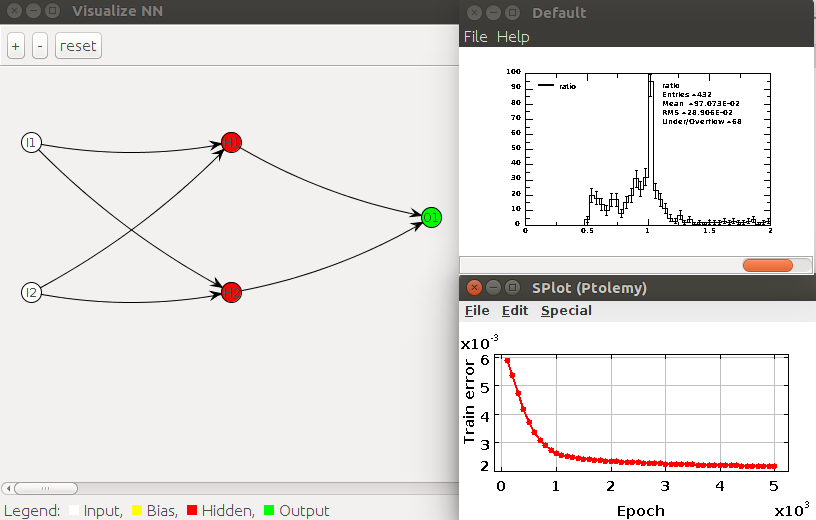## Neural Network using Joone

Joone is another graphical editor and engine for neural networks. This neural net is available asorg.joone.net.NeuralNet. Let us a show example that train neural network, show the global error and validate the network. We use "in memory" data, unlike data which can be read from files.

The global error as a function of epoch is shown below: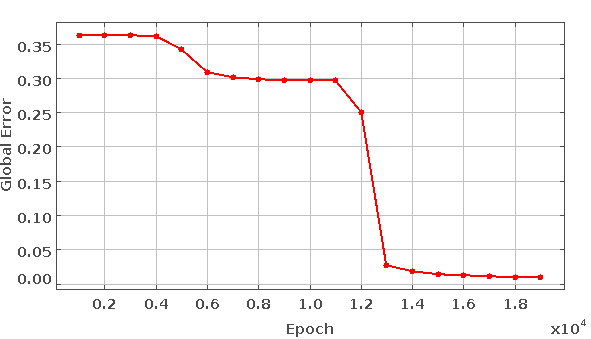## Time series forecast using Joone

Now we will use the Joone Neural Network for forecasting market predictions, using a generated time series in the form cos(x)*sin(x)+Gaussian noise.

Here is the output image that shows the original time series and the predicted trends (n red).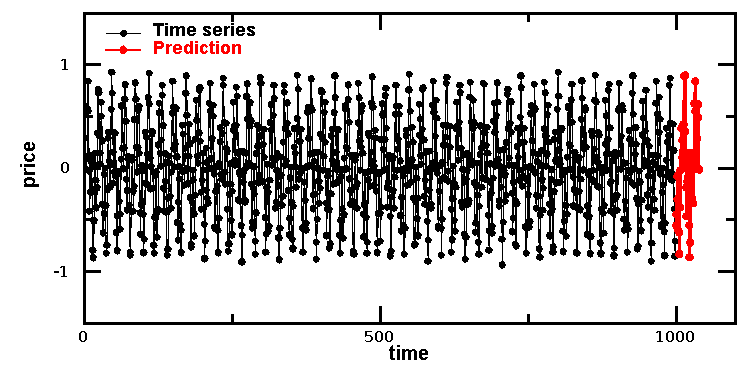As you can see, the neural net correctly predicts the expected behavior.